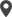Enable Dark Mode!By: Vinaya Suresh

# Hashing Algorithms Using Python

## Technical

Security is a primary concern for all applications. So in Odoo, the passwords are hashed so that they can never be used and modified by an unauthorized person.
The process of converting a string/key to another value is known as hashing. Moreover, the value is generated using a specific mathematical function known as a hash function.
This blog will provide you an insight on hashing algorithms using python.
The hash function helps to store strings such as passwords in the form of hash values so that it becomes impossible for the unauthorized users from decrypting it. In addition, the intruder cannot log in to an application using this hash value.
The generated value is known as the hash. Usually, the length of the string which is converted to hash is smaller than the hash code. Moreover, the hash value is obtained with the help of a hash function, this process of obtaining hash code requires a series of steps. Therefore, the algorithm, which describes how to use the hash functions is known as a hashing algorithm.
Hashing is a unidirectional process which means it is not possible to get the original data from the hash. Additionally, the MD-5(Message-Digest Algorithm 5) and  SHA(Secure Hash Algorithm) family algorithms are the most commonly used hashing algorithms.
Now let's move on to understanding the Message digest algorithm -5 in the next section.

MD5 (Message-Digest Algorithm 5)
MD5 algorithm generates a hash value of 128-bit length. Moreover, the MD5 algorithm can be easily understood by the following example code which generates an MD5 hash for a string in python 3.
`import hashlibresult = hashlib.md5(b"Test password").hexdigest()print(result)`
Output for the code is shown below:
ffe24c9204d65518429aa701f3a6bdc5

The above code generates a hash value ffe24c9204d65518429aa701f3a6bdc5 for string ‘Test password’.
In order to use the hashing algorithm, we need to import hashlib. Here the function b” ” is used to convert the string to bytes. Since the hashing function only accepts bytes. Additionally, the hexdigest() function converts the encoded data to hexadecimal format.
MD5 algorithms are faster compared to SHA but the security of this algorithm is poor compared to the other algorithm.
Now let us see the SHA family algorithms one by one.

SHA256
The SHA256 algorithm is the most commonly used hashing algorithm and is more secure than the MD5 algorithm.
The following example code generates a SHA256 algorithm:
`import hashlibtest_str = "password"result = hashlib.sha256(test_str.encode())print(result.hexdigest()) `
Output:
5e884898da28047151d0e56f8dc6292773603d0d6aabbdd62a11ef721d1542d8

The SHA256 algorithm belongs to the SHA-2 family. Additionally, here encode() function is used to convert string to bytes that is acceptable by the hash function.
The following are the main reasons for using SHA 256 algorithm is that:
- It is more secure than other hashing algorithms
- The collision possibility is less because there are 2256 possibilities for hash value, so the chance of having the same hash value for two different strings is lower.
Let's now move on to understanding the SHA384 algorithm in the next section.

SHA384
The SHA384 algorithm generates a hash value of 384-bit length. The following example code which generates a SHA384 algorithm:
`import hashlibtest_str = "password"result = hashlib.sha384(test_str.encode())print(result.hexdigest())   `
Output:
a8b64babd0aca91a59bdbb7761b421d4f2bb38280d3a75ba0f21f2bebc45583d446c598660
c94ce680c47d19c30783a7
Let's now move on to understanding the SHA224 algorithm in the next section.

SHA224
The SHA224 algorithm generates a hash value of 224-bit length. It belongs to the SHA2 family. The following example code which generates an SHA224 algorithm:
`import hashlibtest_str = "password"result = hashlib.sha224(test_str.encode())print(result.hexdigest()) `
Output:
d63dc919e201d7bc4c825630d2cf25fdc93d4b2f0d46706d29038d01
Let's now move on to understanding the SHA512 algorithm in the next section.

SHA512
The SHA512 algorithm creates a hash value of length 512 bits. It belongs to the SHA2 family.The following example code which generates an SHA512 algorithm:
`import hashlibtest_str = "password"result = hashlib.sha512(test_str.encode())print(result.hexdigest())`
Output:
b109f3bbbc244eb82441917ed06d618b9008dd09b3befd1b5e07394c706a8bb980b1d7785
e5976ec049b46df5f1326af5a2ea6d103fd07c95385ffab0cacbc86
Let's now move on to understanding the SHA-1 algorithm in the next section.

SHA-1
The SHA-1 algorithm creates a 160-bit hash value. The usage of MD5 started to get replaced after the introduction of SHA-1. The below python code generates the hash value for the given string “password”:
`import hashlibtest_str = "password"result = hashlib.sha1(test_str.encode())print(result.hexdigest())`
Output:
5baa61e4c9b93f3f0682250b6cf8331b7ee68fd8
Moreover, the SHA-1 hashing algorithm has vulnerabilities so it is considered to be less resistant to attacks.

In conclusion, the most recommended algorithm to be used is SHA-256 as it generates a hash of length 256 bits. Hashing can also be used to check the integrity of files while transferring digital files. One such algorithm is CRC32(Cyclic Redundancy Check). Moreover, this hashing technique is used to detect accidental file changes. In addition, it is not that commonly used as compared to other hashing techniques. So it can be concluded that a hashing algorithm is considered to be secure if it offers no chance of hash collision thereby providing security.

If you need any assistance in odoo, we are online, please chat with us.

### Recent Posts

#### How to Create a Voice Chatbot Using Openai's APICalicut

Cybrosys Technologies Pvt. Ltd.
Neospace, Kinfra Techno Park
Kakkancherry, Calicut
Kerala, India - 673635London

Cybrosys Limited
Alpha House,
100 Borough High Street, London,
SE1 1LB, United KingdomKochi

Cybrosys Technologies Pvt. Ltd.
1st Floor, Thapasya Building,
Kochi, India - 682030.Bangalore

Cybrosys Techno Solutions
The Estate, 8th Floor,